fractions
mixed numbers
Convert fractions
Multiply
*challenge*
100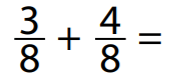100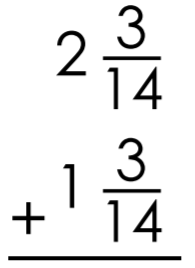100

Write each improper fraction as a mixed number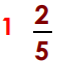100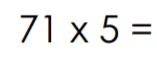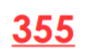100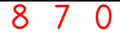200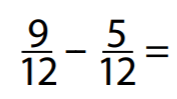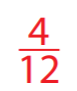200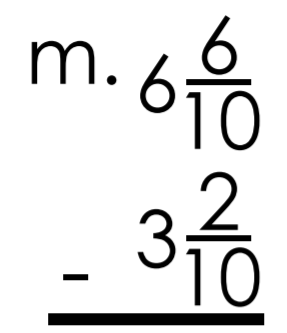200

Write each improper fraction as a mixed number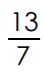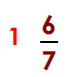200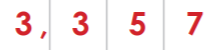200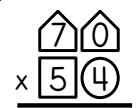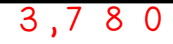300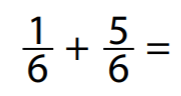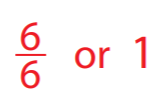300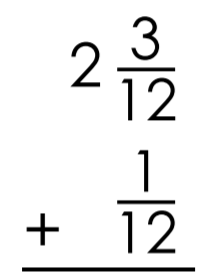300

Write each improper fraction as a mixed number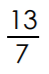300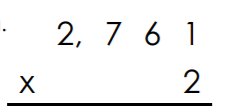300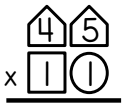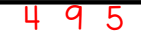400

I'm looking for a VERY SPECIFIC answer: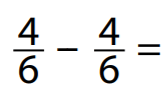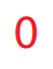400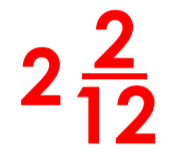400

Write each improper fraction as a mixed number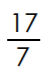400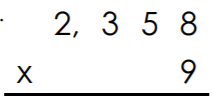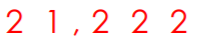400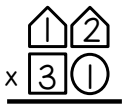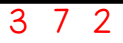500

Two parts to the answer: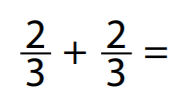500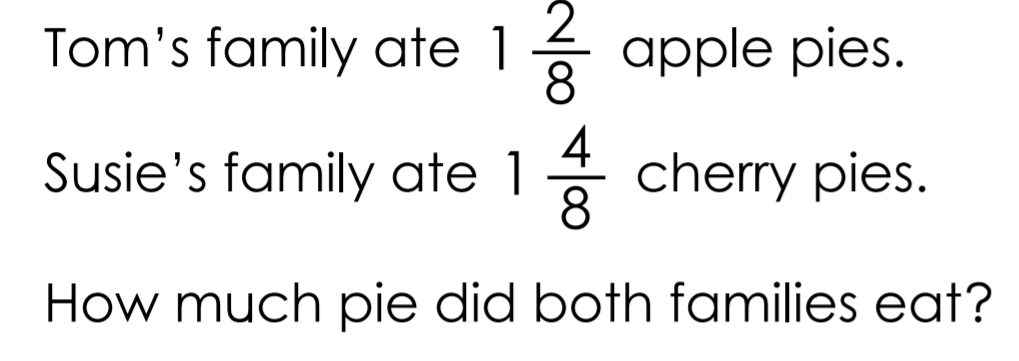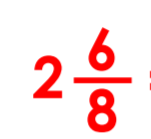500

Mrs. Jones bakes pies. She always cuts each pie into 8 slices. There are 13 slices left on the counter. Write the number of pies on the counter as a mixed number and as an improper fraction.500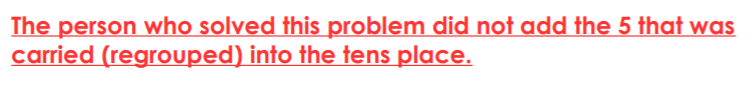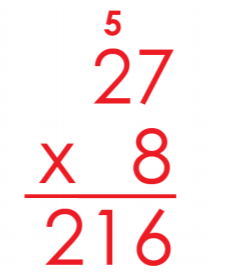500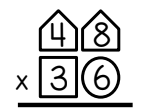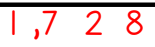Click to zoom